## Rounding Whole Numbers

### Learning Outcomes

• Identify the place value of a digit used for rounding
• Round a whole number to a specific place value

In $2013$, the U.S. Census Bureau reported the population of the state of New York as $19,651,127$ people. It might be enough to say that the population is approximately $20$ million. The word approximately means that $20$ million is not the exact population, but is close to the exact value.

The process of approximating a number is called rounding. Numbers are rounded to a specific place value depending on how much accuracy is needed. Saying that the population of New York is approximately $20$ million means we rounded to the millions place. The place value to which we round to depends on how we need to use the number.

Using the number line can help you visualize and understand the rounding process. Look at the number line below. Suppose we want to round the number $76$ to the nearest ten. Is $76$ closer to $70$ or $80$ on the number line?

We can see that $76$ is closer to $80$ than to $70$. So $76$ rounded to the nearest ten is $80$.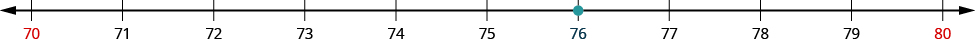Now consider the number $72$. Find $72$ on the number line.

We can see that $72$ is closer to $70$, so $72$ rounded to the nearest ten is $70$.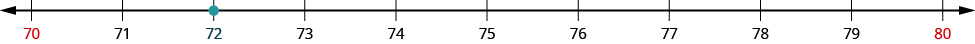How do we round $75$ to the nearest ten?

Find $75$ on the number line.

The number $75$ is exactly midway between $70$ and $80$.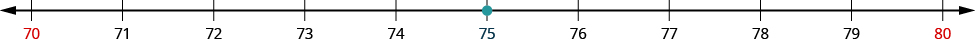So that everyone rounds the same way in cases like this, mathematicians have agreed to round to the higher number, $80$. So, $75$ rounded to the nearest ten is $80$.

Now that we have looked at this process on the number line, we can introduce a more general procedure. To round a number to a specific place, look at the number to the right of that place. If the number is less than $5$, round down. If it is greater than or equal to $5$, round up.

So, for example, to round $76$ to the nearest ten, we look at the digit in the ones place.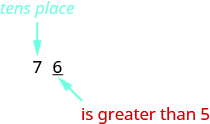The digit in the ones place is a $6$. Because $6$ is greater than or equal to $5$, we increase the digit in the tens place by one. So the $7$ in the tens place becomes an $8$. Now, replace any digits to the right of the $8$ with zeros. So, $76$ rounds to $80$.Let’s look again at rounding $72$ to the nearest $10$. Again, we look to the ones place.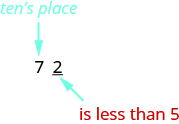The digit in the ones place is $2$. Because $2$ is less than $5$, we keep the digit in the tens place the same and replace the digits to the right of it with zero. So $72$ rounded to the nearest ten is $70$.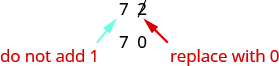### Round a whole number to a specific place value

1. Locate the given place value. All digits to the left of that place value do not change.
2. Underline the digit to the right of the given place value.
3. Determine if this digit is greater than or equal to $5$.
• Yes—add $1$ to the digit in the given place value.
• No—do not change the digit in the given place value.
4. Replace all digits to the right of the given place value with zeros.

### TIP

If the digit to the right of your given place value is 5 or above, give your given place value a shove.

If the digit to the right of your given place value is 4 or less, let your given place value rest.

### example

Round $843$ to the nearest ten.

Solution

 Locate the tens place.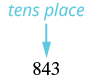Underline the digit to the right of the tens place.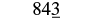Since $3$ is less than $5$, do not change the digit in the tens place.Replace all digits to the right of the tens place with zeros.Rounding $843$ to the nearest ten gives $840$.

### Example

Round each number to the nearest hundred:

1. $23,658$
2. $3,978$

### example

Round each number to the nearest thousand:

1. $147,032$
2. $29,504$

### try it

Watch the video below for more examples of how to round whole numbers to a given place value.

## Contribute!

Did you have an idea for improving this content? We’d love your input.